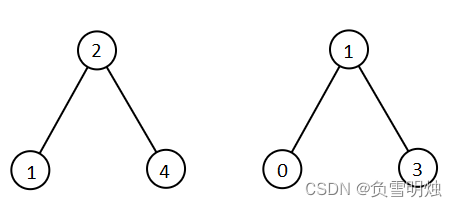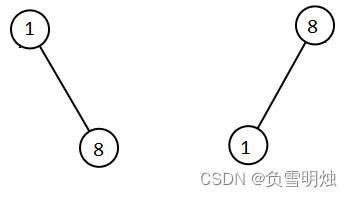# 1305. All Elements in Two Binary Search Trees 两棵二叉搜索树中的所有元素

@TOC

## # 题目描述输入：root1 = [2,1,4], root2 = [1,0,3]输入：root1 = [1,null,8], root2 = [8,1]



• 每棵树的节点数在 [0, 5000] 范围内
• -105 <= Node.val <= 105

## # 解题方法

### # 方法一：中序遍历 + 归并

LeetCode 上也有相关题目：

# Definition for a binary tree node.
# class TreeNode(object):
#     def __init__(self, val=0, left=None, right=None):
#         self.val = val
#         self.left = left
#         self.right = right
class Solution(object):
def getAllElements(self, root1, root2):
"""
:type root1: TreeNode
:type root2: TreeNode
:rtype: List[int]
"""
nums1 = []
nums2 = []
self.inOrder(root1, nums1)
self.inOrder(root2, nums2)
return self.merge(nums1, nums2)

def inOrder(self, root, nums):
if not root:
return
self.inOrder(root.left, nums)
nums.append(root.val)
self.inOrder(root.right, nums)

def merge(self, nums1, nums2):
res = []
p1 = 0
p2 = 0
while p1 < len(nums1) and p2 < len(nums2):
if nums1[p1] < nums2[p2]:
res.append(nums1[p1])
p1 += 1
else:
res.append(nums2[p2])
p2 += 1
if p1 < len(nums1):
res.extend(nums1[p1:])
if p2 < len(nums2):
res.extend(nums2[p2:])
return res


• 时间复杂度：
• 空间复杂度：

## # 总结

1. 本题考察了三个知识点：BST 的性质、中序遍历、合并两个有序数组。都是基础问题，可见基础知识的重要性，一通百通。

## # 日期

2022 年 5 月 1 日—— 劳动人民的假日，从刷题开始！